• 多目标遗传算法应用案例，简单易懂易上手。其中还有清晰的案例。
•  新的个性化教学机制，结合非支配排序理念和TLBO的教学过程。  INM-TLBO指令机制的基本概念、实现过程及其功能。  INM-TLBO 对三个测试问题集（两个或三个目标）进行了评估。 将数值结果与其他最先进算法的...matlab
• 针对多目标资源受限项目调度的特性, 基于结合活动列表和资源列表的编码设计了合理的交叉操作, 提出一种多目标教学算法. 为了在个体间有效交互信息, 在教师阶段非支配个体作为教师与学生执行交叉, 而在学生阶段学生间...
• 文章目录第三十二章 分组教学优化算法（Group teaching optimization algorithm,GTOA）基本思想GTOA框架能力分组阶段教师阶段学生阶段教师分配阶段GTOA实现 第三十二章 分组教学优化算法（Group teaching ...
获取更多资讯，赶快关注公众号(名称：智能制造与智能调度，公众号：deeprlscheduler)吧！

文章目录
第三十二章 分组教学优化算法（Group teaching optimization algorithm,GTOA）基本思想GTOA框架能力分组阶段教师阶段学生阶段教师分配阶段
GTOA实现

第三十二章 分组教学优化算法（Group teaching optimization algorithm,GTOA）
很多传统的智能优化算法往往需要很多额外的控制参数，而每个参数设置都需要一定的先验知识，或不断尝试，一旦这些参数设置的不合适，就会严重影响算法的优化性能。为了解决这样的问题，天津大学的Yiying Zhang于2020年提出了一种新的全局优化算法——分组教学优化算法(Group teaching optimization algorithm,GTOA)。
该算法受到分组教学机制的启发。分组教学是一种常见的教学模式，可以简述如下:学生首先按照规定的规则被分成若干组，然后结合每个小组的特点，教师会运用具体的教学方法来提高小组的知识。
基本思想
在中国历史上，孔子最早提出了"因材施教"的教育理念，就是说教师应该根据不同学生的特点制定适合他们的教学方法，所以GTOA的思想在于通过模仿分组教学来提升整个班级的知识水平。考虑到学生之间的各种差异，分组教学在实践中实施起来比较复杂。为了使分组教学适应于作为一种优化技术，我们首先假设种群、决策变量和适应度值分别与学生、提供给学生的课程和学生的知识类似。在此基础上，定义了4条规则，构建了一个简单且不失一般性的分组教学模型：
学生之间的唯一区别在于接受知识的能力，学生接受知识的能力差异越大，教师在制定教学计划时面临的挑战就越大。一个好的教师往往更关注接受知识能力较差的学生，而不是接受知识能力较强的学生。在课余时间，学生可以通过自学和与其他学生的交流来获得自己的知识。良好的教师分配机制对提高学生的知识水平非常有帮助。
GTOA框架
GTOA的分组教学模型中存在4个阶段，包括教师分配阶段、能力分组阶段、教师阶段和学生阶段，如下图所示。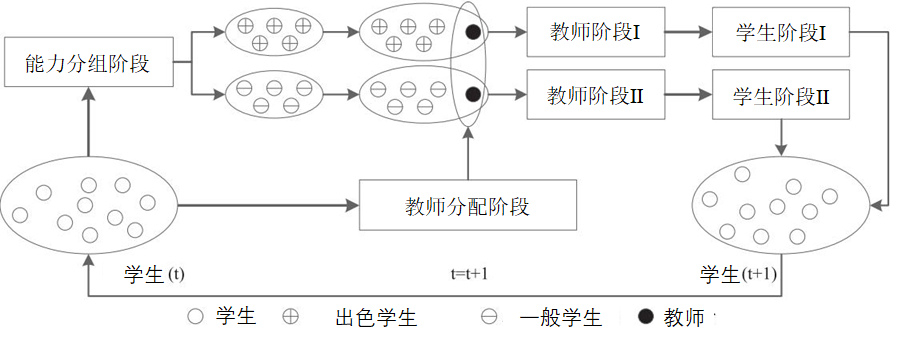能力分组阶段
不失一般性，可以假设整个班级的知识服从正太分布：

f

(

x

)

=

1

2

π

δ

e

−

(

x

−

u

)

2

2

δ

2

(1)

f(x)=\frac{1}{\sqrt{2 \pi} \delta} e^{\frac{-(x-u)^{2}}{2 \delta^{2}}}\tag{1}

其中

u

u

为整个班级的平均知识水平，

δ

\delta

为标准差。一位优秀的教师不仅要考虑如何提高平均知识

u

u

，而且要考虑如何减少标准差

δ

\delta

。为了为了实现这一目标，教师应该针对其学生制定合适的教学计划。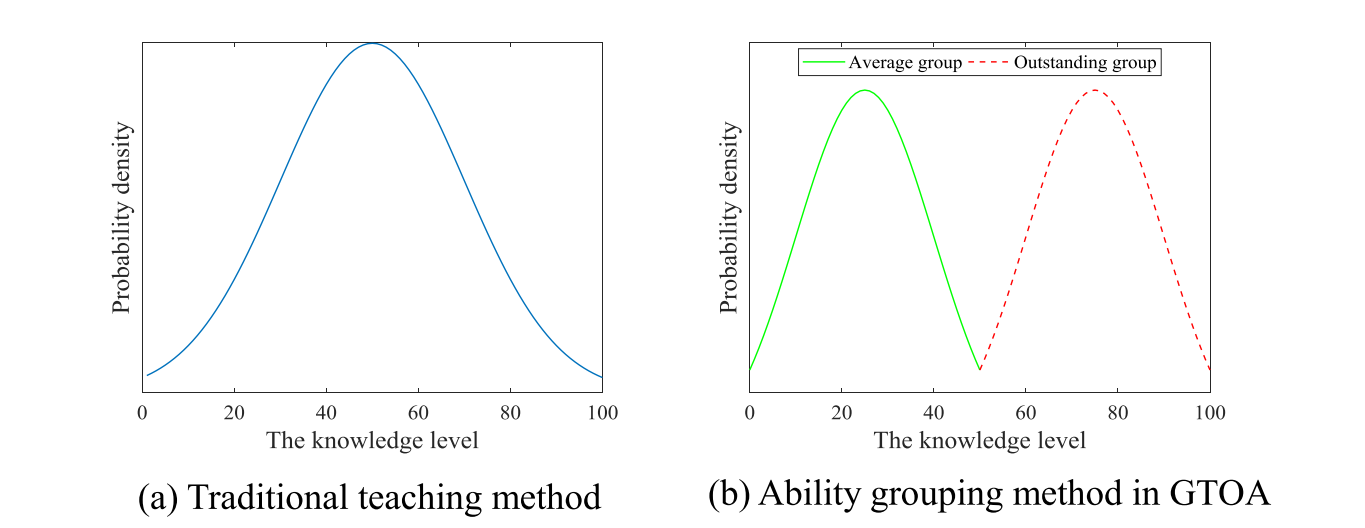上图为两种不同教学方法的分布模型，图(a)为传统教学方法的知识分布，可以看出该分布相对分散。为了更好地表现分组教学的特点，可以将所有学生根据接受知识的水平分成两组，这两个组在GTOA中同等重要，因而这两组具有相同的学生数量。接受能力强的学生称为出色学生或优秀学生，而接受能力差一点的则称为一般学生或平庸学生。图(b)展示了基于能力分组的知识分布，可以看出出色组和一般组的标准差比(a)中的小很多。考虑到第一条规则，由于标准差变小，教师在制定教学计划时更容易采用能力分组法，而不是传统的教学方法。值得注意的是，在图(b)中，出色组和一般组的标准差可能会随着教学活动的进行而增大。为了解决这个问题，能力分组在GTOA中是一个动态的过程，需要每当在一个学习周期结束之后再进行一次分组。
教师阶段
教师阶段意味着学生从教师那学习知识，这对应于第二条规则。在GTOA中，教师为一般组和出色组制定不同的教学计划。
教师阶段Ⅰ：由于接受知识的能力较强，在提出的GTOA中，教师更加注重对出色组整体知识的提高。更具体地说，教师可以尽其所能提高全班的平均知识水平。此外，还需要考虑学生在接受知识方面的差异。因此，出色组的学生可以通过下式获取知识：

x

teacher

,

i

t

+

1

=

x

i

t

+

a

×

(

T

t

−

F

×

(

b

×

M

t

+

c

×

x

i

t

)

)

(2)

\mathbf{x}_{\text {teacher }, i}^{t+1}=\mathbf{x}_{i}^{t}+a \times\left(\mathbf{T}^{t}-F \times\left(b \times \mathbf{M}^{t}+c \times \mathbf{x}_{i}^{t}\right)\right)\tag{2}

M

t

=

1

N

∑

i

=

1

N

x

i

t

(3)

\mathbf{M}^{t}=\frac{1}{N} \sum_{i=1}^{N} \mathbf{x}_{i}^{t}\tag{3}

b

+

c

=

1

(4)

b+c=1\tag{4}

其中

t

t

为当前迭代次数，

N

N

是学生数量，

x

i

t

\mathbf{x}_{i}^{t}

为学生

i

i

在时刻

t

t

的知识，

T

t

\mathbf{T}^{t}

是教师在时刻

t

t

的知识，

M

t

\mathbf{M}^{t}

是时刻

t

t

小组的平均知识，

F

F

是决定教师教学成果的教学因子，

x

teacher

,

i

t

+

1

\mathbf{x}_{\text {teacher }, i}^{t+1}

是学生

i

i

在时刻

t

t

向其老师学习到的知识。

a

,

b

,

c

a,b,c

均是

[

0

,

1

]

[0,1]

内的随机数。

F

F

的值通常为1或2。
教师阶段Ⅱ：考虑到接受知识的能力较差，根据第二条规则，教师更关注一般组，教师倾向于从个体的角度提高学生的知识水平。因此一般组的学生可以通过下式获取知识：

x

teacher

,

i

t

+

1

=

x

i

t

+

2

×

d

×

(

T

t

−

x

i

t

)

(5)

\mathbf{x}_{\text {teacher }, i}^{t+1}=\mathbf{x}_{i}^{t}+2 \times d \times\left(\mathbf{T}^{t}-\mathbf{x}_{i}^{t}\right)\tag{5}

其中

d

d

为

[

0

,

1

]

[0,1]

内的随机数。
此外，学生并不能保证在教师阶段一定会获得知识，以最小化问题为例，只有当适应度值降低时才会更新下一时刻的位置。

x

teacher

,

i

t

+

1

=

{

x

teacher

,

i

t

+

1

,

f

(

x

teacher

,

i

t

+

1

)

<

f

(

x

i

t

)

x

i

t

,

f

(

x

teacher,

,

i

t

+

1

)

≥

f

(

x

i

t

)

(6)

\mathbf{x}_{\text {teacher }, i}^{t+1}=\left\{\begin{array}{l} \mathbf{x}_{\text {teacher }, i}^{t+1}, f\left(\mathbf{x}_{\text {teacher }, i}^{t+1}\right)<f\left(\mathbf{x}_{i}^{t}\right) \\ \mathbf{x}_{i}^{t}, f\left(\mathbf{x}_{\text {teacher, }, i}^{t+1}\right) \geq f\left(\mathbf{x}_{i}^{t}\right) \end{array}\right.\tag{6}

学生阶段
根据第三条规则，学生阶段也包括Ⅰ和Ⅱ两个阶段。在课余时间，学生可以有两种不同的方式获取知识：自学和与同学交流，可通过下式表达：

x

student

,

i

t

+

1

=

{

x

teacher

,

i

t

+

1

+

e

×

(

x

teacher

,

i

t

+

1

−

x

teacher

,

j

t

+

1

)

+

g

×

(

x

teacher

,

i

t

+

1

−

x

i

t

)

,

f

(

x

teacher

,

i

t

+

1

)

<

f

(

x

teacher

,

j

t

+

1

)

x

teacher

,

i

t

+

1

−

e

×

(

x

teacher

,

i

t

+

1

−

x

teacher

,

j

t

+

1

)

+

g

×

(

x

teacher

,

i

t

+

1

−

x

i

t

)

,

f

(

x

teacher

,

i

t

+

1

)

≥

f

(

x

teacher

,

j

t

+

1

)

(7)

\mathbf{x}_{\text {student }, i}^{t+1}=\left\{\begin{array}{l} \mathbf{x}_{\text {teacher }, i}^{t+1}+e \times\left(\mathbf{x}_{\text {teacher }, i}^{t+1}-\mathbf{x}_{\text {teacher }, j}^{t+1}\right) +g \times\left(\mathbf{x}_{\text {teacher }, i}^{t+1}-\mathbf{x}_{i}^{t}\right), f\left(\mathbf{x}_{\text {teacher }, i}^{t+1}\right)<f\left(\mathbf{x}_{\text {teacher }, j}^{t+1}\right) \\ \mathbf{x}_{\text {teacher }, i}^{t+1}-e \times\left(\mathbf{x}_{\text {teacher }, i}^{t+1}-\mathbf{x}_{\text {teacher }, j}^{t+1}\right)+g \times\left(\mathbf{x}_{\text {teacher }, i}^{t+1}-\mathbf{x}_{i}^{t}\right), f\left(\mathbf{x}_{\text {teacher }, i}^{t+1}\right) \geq f\left(\mathbf{x}_{\text {teacher }, j}^{t+1}\right) \end{array}\right.\tag{7}

其中

e

e

和

g

g

为两个

[

0

,

1

]

[0,1]

之间的随机数。

x

student

,

i

t

+

1

\mathbf{x}_{\text {student }, i}^{t+1}

是学生

i

i

在时刻

t

t

通过学生阶段学习到的知识，

x

teacher

,

j

t

+

1

\mathbf{x}_{\text {teacher }, j}^{t+1}

是学生

j

j

在时刻

t

t

通过老师阶段学习到的知识，学生

j

∈

{

1

,

2

,

.

.

.

,

i

−

1

,

i

+

1

,

.

.

.

,

N

}

j\in\{1,2,...,i-1,i+1,...,N\}

是随机选择的。上式中的第2项和第3项分别表示向其他同学学习和自学。
同样，学生也并不能保证在学生阶段一定会获得知识，以最小化问题为例，只有当适应度值降低时才会更新下一时刻的位置。

x

i

t

+

1

=

{

x

teacher

,

i

t

+

1

,

f

(

x

teacher

,

i

t

+

1

)

<

f

(

x

student

,

i

t

+

1

)

x

student

,

i

t

+

1

,

f

(

x

teacher

,

i

t

+

1

)

≥

f

(

x

student

,

i

t

+

1

)

(8)

\mathbf{x}_{i}^{t+1}=\left\{\begin{array}{l} \mathbf{x}_{\text {teacher }, i}^{t+1}, f\left(\mathbf{x}_{\text {teacher }, i}^{t+1}\right)<f\left(\mathbf{x}_{\text {student }, i}^{t+1}\right) \\ \mathbf{x}_{\text {student }, i}^{t+1}, f\left(\mathbf{x}_{\text {teacher }, i}^{t+1}\right) \geq f\left(\mathbf{x}_{\text {student }, i}^{t+1}\right) \end{array}\right.\tag{8}

其中

x

i

t

+

1

\mathbf{x}_{i}^{t+1}

是学生

i

i

一次学习循环后在时刻

t

+

1

t+1

的知识。
教师分配阶段
基于第四条规则，如何制定一个良好的教师配置机制，对提高学生的知识水平具有十分重要的意义。模仿灰狼优化中的保留三个最优解的思想，教师分配机制可以表达为：

T

t

=

{

x

first

t

,

f

(

x

first

t

)

≤

f

(

x

firs

t

+

x

sscond

t

+

x

third

t

3

)

x

first

t

+

x

second

t

+

x

third

t

3

,

f

(

x

first

t

)

>

f

(

x

firs

t

+

x

second

t

+

x

thicd

t

3

)

(9)

\mathbf{T}^{t}=\left\{\begin{array}{ll} \mathbf{x}_{\text {first }}^{t}, & f\left(\mathbf{x}_{\text {first }}^{t}\right) \leq f\left(\frac{\mathbf{x}_{\text {firs }}^{t}+\mathbf{x}_{\text {sscond }}^{t}+\mathbf{x}_{\text {third }}^{t}}{3}\right) \\ \frac{\mathbf{x}_{\text {first }}^{t}+\mathbf{x}_{\text {second }}^{t}+\mathbf{x}_{\text {third }}^{t}}{3}, & f\left(\mathbf{x}_{\text {first }}^{t}\right)>f\left(\frac{\mathbf{x}_{\text {firs }}^{t}+\mathbf{x}_{\text {second }}^{t}+\mathbf{x}_{\text {thicd }}^{t}}{3}\right) \end{array}\right.\tag{9}

其中

x

f

i

r

s

t

t

,

x

s

e

c

o

n

d

t

,

x

t

h

i

r

d

t

\bf{x}_{{\rm{first }}}^t,\bf{x}_{{\rm{second }}}^t,\bf{x}_{{\rm{third }}}^t

分别为第一优、第二优和第三优学生，为了加快算法的收敛，出色组和一般组共用相同的老师。
GTOA实现
下面给出GTOA的实现步骤。
Step1：初始化
(1.1)初始化参数。这些参数包括最大评估次数

T

m

a

x

T_{max}

，当前评估次数

T

c

u

r

r

e

n

t

(

T

c

u

r

r

e

n

t

=

0

)

T_{current}(T_{current}=0)

，种群大小

N

N

，决策变量的上下界

u

\bf{u}

和

l

\bf{l}

，维度

D

D

和适应度函数

f

(

⋅

)

f(·)

。
(1.2)初始化种群。根据以上初始参数随机生成种群

X

t

\bf{X}^t

:

X

t

=

[

x

1

t

,

x

2

t

,

…

,

x

N

t

]

T

=

[

x

1

,

1

t

x

1

,

2

t

…

x

1

,

D

t

x

2

,

1

t

x

2

,

2

t

…

x

2

,

D

t

⋮

⋮

⋮

x

N

,

1

t

x

N

,

2

t

…

x

N

,

D

t

]

(10)

\mathbf{X}^{t}=\left[\mathbf{x}_{1}^{t}, \mathbf{x}_{2}^{t}, \ldots, \mathbf{x}_{N}^{t}\right]^{\mathrm{T}}=\left[\begin{array}{cccc} x_{1,1}^{t} & x_{1,2}^{t} & \dots & x_{1, D}^{t} \\ x_{2,1}^{t} & x_{2,2}^{t} & \dots & x_{2, D}^{t} \\ \vdots & \vdots & & \vdots \\ x_{N, 1}^{t} & x_{N, 2}^{t} & \dots & x_{N, D}^{t} \end{array}\right]\tag{10}

x

i

,

j

t

=

l

i

+

(

u

i

−

l

i

)

×

κ

(11)

x_{i, j}^{t}=l_{i}+\left(u_{i}-l_{i}\right) \times \kappa\tag{11}

其中

κ

\kappa

为

[

0

,

1

]

[0,1]

之间的随机数。
Steo2：种群评估。
计算个体的适应度值，选出最优解

G

t

\mathbf{G}^{t}

，更新当前函数评估次数

T

c

u

r

r

e

n

t

T_{current}

：

T

c

u

r

r

e

n

t

=

T

c

u

r

r

e

n

t

+

N

(12)

T_{current}=T_{current}+N\tag{12}

Step3：终止准则
如果当前评估次数

T

c

u

r

r

e

n

t

T_{current}

大于最大评估次数

T

m

a

x

T_{max}

，终止算法并输出最优解

G

t

\mathbf{G}^{t}

，否则跳转至Step4。
Step4：教师分配阶段
选出三个最优解，根据公式(9)计算

T

t

{{\bf{T}}^t}

。
Step5：能力分组阶段
基于适应度值将种群分成两个组，最好的一半个体组成出色组

X

good

t

\mathbf{X}_{\text {good }}^{t}

，剩余的个体组成一般组

X

t

。这两组共用相同的老师。
Step6：教师阶段和学生阶段
(6.1)对于

X

good

t

\mathbf{X}_{\text {good }}^{t}

组，基于公式(2),(3),(4)和(6)实现教师阶段，再根据(7)和(8)执行学生阶段。最终获取新的

X

good

t

+

1

\mathbf{X}_{\text {good }}^{t+1}

。
(6.2)对于

X

t

组，基于公式(5)和(6)实现教师阶段，再根据(7)和(8)执行学生阶段。最终获取新的

X

t

+

1

。
Step7：构建种群
将

X

good

t

+

1

\mathbf{X}_{\text {good }}^{t+1}

和

X

t

+

1

组成一个新的种群

X

t

+

1

\mathbf{X}^{t+1}

。
Step8：种群评估
计算个体的适应度值，选出最优解

G

t

\mathbf{G}^{t}

，更新当前函数评估次数

T

c

u

r

r

e

n

t

T_{current}

：

T

c

u

r

r

e

n

t

=

T

c

u

r

r

e

n

t

+

2

N

+

1

(13)

T_{current}=T_{current}+2N+1\tag{13}

然后执行Step3。
下图为上述过程的流程图。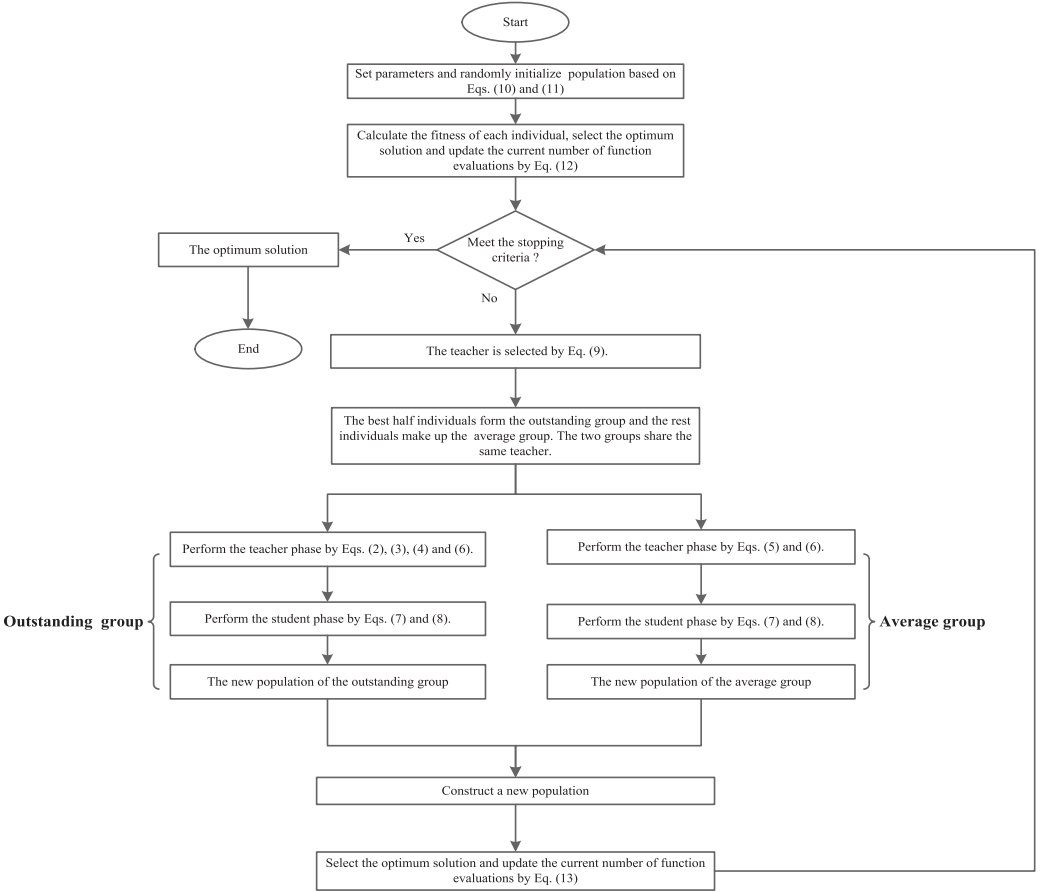展开全文群体智能优化
• 目录（这是一篇半年前写的东西……）摘要背景算法学生初始化教学阶段学习阶段流程总结优缺点优点缺点一些改进总结参考...本文对教学优化算法进行简单介绍，并对教学优化算法在各方面的一些改进扩展进行描述。 背景 近


目录
摘要背景算法学生初始化教学阶段学习阶段流程总结
优缺点优点缺点
一些改进总结参考文献

摘要
教学优化算法（Teaching-learning-based optimization, TLBO）是一种基于种群的启发式随机群智能算法。与其他的进化算法类似，该方法也存在迭代过程。该过程分为两步，每个阶段执行各自的优化。相比于其他的算法，教学优化算法的主要优势在于概念简单、超参数量少、收敛快速。本文对教学优化算法进行简单介绍，并对教学优化算法在各方面的一些改进扩展进行描述。
背景
近年来，随着各种领域的优化问题不断增多，更多的优化方法被提出以适用于各类问题。在早期，大多数优化算法主要关注数学技术。而这些方法的主要问题在于容易陷入局部最优解而无法找到最优解。而最近，各种启发式算法成为了热点，这其中包括如遗传算法、演化规划、演化策略、遗传规划等所属的进化算法，蚁群算法、粒子群算法等所属的群智能算法，以及引力搜索算法、人工水滴算法等所属的非生物学元启发式算法。然而这些方法中，除了种群大小和迭代次数，往往还存在一些其他需要设定的超参数。合理设置和适当调整这些特定于算法的超参数，对于这些算法的搜索能力至关重要。因此，希望能开发没有算法特定超参数的优化算法。Rao等人提出了基于教学学习的优化算法[2-3]，它的灵感来自于课堂教学过程，模仿教师对学生的影响。与其他群体智能算法类似，教学优化算法基于种群的启发式随机优化算法，但它的优点在于不需要任何特定于算法的超参数。
算法
在教学优化算法中，学生被当作是分布在决策变量空间中的搜索点，其中，最优的学生被定义为该班级的教师。与传统的进化算法和群智能算法不同的是教学优化算法的迭代进化过程包括教学阶段和学习阶段，如图1所示。在教学阶段，为了提高班级的平均水平，学生会通过向教师学习来提高自身水平；之后在学习阶段，会通过与随机选择的另一位学生进行互动学习来提高自身水平。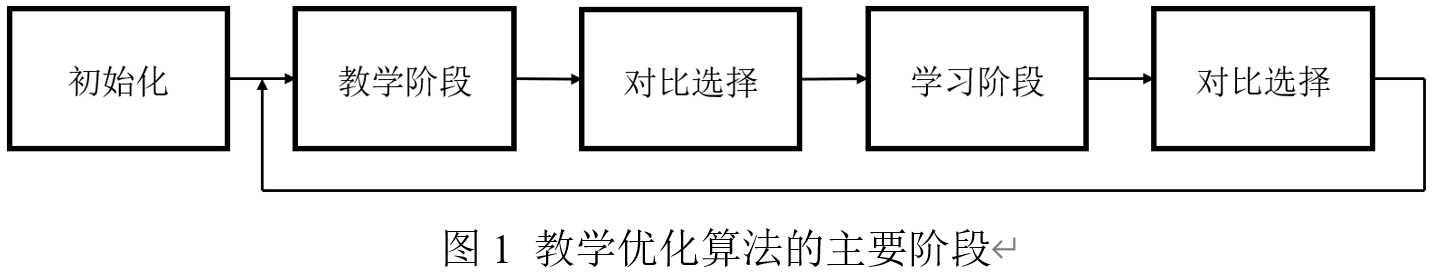学生初始化
记决策变量维度为

D

D

，第

i

i

个学生（搜索点）表示为

X

i

=

(

x

i

1

,

x

i

2

,

…

,

x

i

D

)

X_i=(x_{i1},x_{i2},…,x_{iD})

，

f

(

X

i

)

f(X_i)

表示为适应度函数，目标是尽可能接近该函数的极小值。

N

N

表示学生总量。班级中第

i

i

个学生

X

i

=

(

x

i

1

,

x

i

2

,

…

,

x

i

D

)

X_i=(x_{i1},x_{i2},…,x_{iD})

可以被初始化为：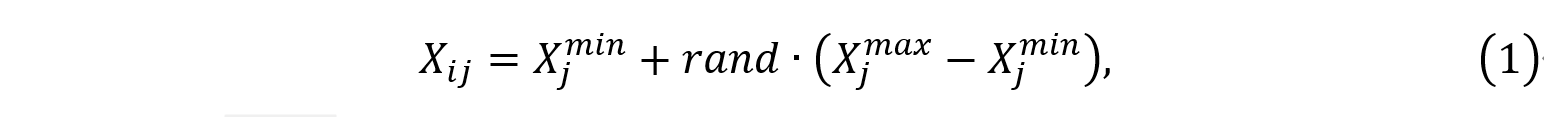其中

X

j

m

a

x

X_j^{max}

和

X

j

m

i

n

X_j^{min}

分别为第j维决策变量的上下界，rand是[0,1]之间的随机数。
教学阶段
在教学阶段，模拟学生通过学习教师与班级平均水平的差异来提高自身水平。对于班级中的第i个学习者

X

i

X_i

，更新机制表示如下：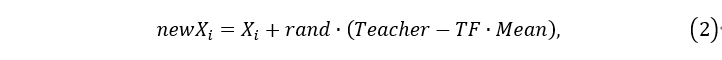其中，

n

e

w

X

i

newX_i

是学生

X

i

X_i

的新取值，

T

e

a

c

h

e

r

Teacher

是当前最优值的学生，并且

M

e

a

n

=

1

/

N

∑

i

=

1

N

X

i

Mean=1/N \sum_{i=1}^N X_i

是班级的平均状态。

T

F

=

r

o

u

n

d

[

1

+

r

a

n

d

(

0

,

1

)

]

TF=round[1+rand(0,1)]

是一个教学因子，决定了要改变的均值的大小。

r

a

n

d

rand

是随机向量，其每个元素都是

[

0

,

1

]

[0,1]

范围内的随机数。在教学阶段之后，学生的值将采用其原先适应度和新适应度之间较小的，然后进入学习阶段。
学习阶段
在学习阶段，模拟学生之间相互讨论、展示和交流的方式进行学习，以提高自身水平。对于学习者

X

i

X_i

，更新公式为：其中，

n

e

w

X

i

newX_i

是学生

X

i

X_i

的新取值，

X

k

X_k

是从班级中随机选取的另一个与

X

i

X_i

不同的学生，

f

(

X

i

)

f(X_i )

和

f

(

X

k

)

f(X_k )

分别是学生

X

i

X_i

和

X

k

X_k

的适应度。

r

a

n

d

rand

是随机向量，其每个元素都是

[

0

,

1

[0,1

]范围内的随机数。和教学阶段类似，学生的值将采用其原先适应度和新适应度之间较小的，然后进入下一轮的教学阶段。
流程总结
整个算法流程总结如下：
输入：初始化

N

N

（学生数量）和

D

D

（决策变量维度）输出：教师

X

T

e

a

c

h

e

r

X_{Teacher}

Step1 初始化所有的学生，并评估适应度函数值。Step2 令适应度函数值最小的学生为

X

T

e

a

c

h

e

r

X_{Teacher}

，计算所有学生的均值

X

M

e

a

n

X_{Mean}

.Step3 当满足终止条件（通常为最大轮次）时，直接退出，输出结果;否则继续向下执行。Step4 对于每一个学生，首先计算

T

F

=

r

o

u

n

d

(

1

+

r

a

n

d

(

0

,

1

)

)

TF=round(1+rand(0,1))

;再根据公式（2）计算学生的新适应度函数值；如果新适应度函数值低于原适应度函数值，则更新学生的值。Step5 对于每一个学生，随机选取另一个与之不同的学生，用公式（3）计算学生的新适应度；如果新适应度函数值低于原适应度函数值，则更新学生的值。Step6 更新

X

T

e

a

c

h

e

r

X_{Teacher}

和

X

M

e

a

n

X_{Mean}

，重复执行Step3. 注意，上述过程中没有考虑到有约束的情况。对于有约束问题，可以采用多种类型的约束处理技术，例如静态罚函数、动态罚函数、自适应罚函数等，也有一些文献[4-5]对教学优化问题进行改进，使其适用于各种有约束问题。
优缺点
优点
教学优化算法属于全局优化算法，与遗传算法和粒子群算法类似，在整个解空间进行搜索，可以达到全局最优值。而且相比于粒子群算法，教学优化算法简化了每一轮内的信息共享机制，所有进化的个体可以更快收敛到全局最优解。学习阶段具有并行性，各个学生之间的学习是一种随机的并行关系，加快了全局搜索的速度。算法简易，超参数少，相比于遗传算法和粒子群算法，教学优化算法所需要的超参数只有种群数量，不涉及到优化过程本身。从而简化了优化的初始设置过程。
缺点
记忆能力较差。最初的解集在搜索过程中将会改变，不能很好地保持种群多样性。教学优化算法的理论基础薄弱。对于其中存在的如多样性过早丢失之类的缺点，缺乏理论的验证。缺少收敛分析方法，同属于全局优化算法的遗传算法和粒子群算法都有比较成熟的方法判定收敛，而教学优化算法很难准确估计收敛速度。在教学阶段具有固有的原点偏差，也即该算法有向原点附近搜索的倾向性。当解决一些远离原点的优化问题时，该算法通常会失去优势。有些文献基于这一点对教学优化算法进行改进。
一些改进
教学优化算法的改进和变种非常多，下面介绍一些常用改进方案。 在最初的教学优化算法中，只有一个教师进行教学，当学生的适应度函数值普遍较高时，教学阶段所带来的效果不够明显；而当学生的适应度函数值普遍较低时，收敛速度又会降低。所以改进的教学优化算法提高了教师的教学能力，通过改进，根据学生的适应度函数值高低将班级划分为多个小班级，每个小班级选取一名教师进行该小班级的教学。进一步，这种多个小班级，可以采用K均值聚类的方式实现。 此外，最初的教学优化算法中教学因子只能是1或2，能力过分单一，影响搜索的能力，容易走入极端的学习状态。所以改进了其中的教学因子部分，使其变为一个自适应的连续值，其表达式为

T

F

=

T

F

m

a

x

−

T

F

m

a

x

−

T

F

m

i

n

i

t

e

r

m

a

x

i

t

e

r

TF=TF_{max}-\frac{TF_{max}-TF_{min}}{iter_{max} }iter

，其中

T

F

m

a

x

TF_{max}

和

T

F

m

i

n

TF_{min}

分别为

T

F

TF

的最大值和最小值，

i

t

e

r

m

a

x

iter_{max}

为最大迭代次数。这种方式与粒子群算法中的线性递减惯性权重类似，能在搜索初期加快迭代的步伐，而在后期进行稳定的搜索。 如果采用小班级教学时，在学习阶段，由于每个小班级中的成员与其他成员相似度可能会比较高，所以可以考虑采用和其他班级成员进行交互学习，提升学习阶段的效果。这种方法还能在一定程度上避免算法陷入局部极小值，保存了样本多样性。
总结
本文对教学优化算法进行介绍，并通过三种函数样例测试该算法的优化效果。教学优化算法由于其自身简单、易收敛的优势，在应用领域经常会被用到。不过该算法在一些场景下仍然存在不足，研究人员基于这些问题也开发出许多不同的变体，以提高其优化性能，并已成功应用于各种优化领域。除此之外，对教学优化算法的收敛特性和动力学进行理论分析仍然是十分有必要的。
参考文献
 Luke S. Essentials of Metaheuristics. Lulu, 2013[J].  Rao R V, Savsani V J, Vakharia D P. Teaching–learning-based optimization: a novel method for constrained mechanical design optimization problems[J]. Computer-Aided Design, 2011, 43(3): 303-315.  Rao R V, Savsani V J, Vakharia D P. Teaching–learning-based optimization: an optimization method for continuous non-linear large scale problems[J]. Information sciences, 2012, 183(1): 1-15.  Yu K, Wang X, Wang Z. Constrained optimization based on improved teaching–learning-based optimization algorithm[J]. Information Sciences, 2016, 352: 61-78.  Rao R V, Savsani V J, Balic J. Teaching–learning-based optimization algorithm for unconstrained and constrained real-parameter optimization problems[J]. Engineering Optimization, 2012, 44(12): 1447-1462.  黄祥东. 教与学优化算法的研究与应用[D].中国矿业大学,2016.  Shi Y, Eberhart R C. Empirical study of particle swarm optimization[C]//Proceedings of the 1999 Congress on Evolutionary Computation-CEC99 (Cat. No. 99TH8406). IEEE, 1999, 3: 1945-1950.  平良川,孙自强.教学优化算法的改进及应用[J].计算机工程与设计,2018,39(11):3531-3537.
展开全文人工智能
• 新高考模式下遗传算法在排课问题中的应用 背景： 随着新高考改革在各个省份的推行，提出了“3+3模式”，即高中阶段的学生，不再区分文理科目，学生可以自主的从政治、历史、地理、物理、化学、生物和技术这7门课程里...
新高考模式下遗传算法在排课问题中的应用
背景： 随着新高考改革在各个省份的推行，提出了“3+3模式”，即高中阶段的学生，不再区分文理科目，学生可以自主的从政治、历史、地理、物理、化学、生物和技术这7门课程里任选3门作为高考的考试科目。   学校将班级分为行政班与教学班两类，行政班则于传统教学模式的班级相同，教学班是为不同行政班学生上选修课程组成的临时班级。一个学生的课程由行政班课程和选修课程组成，行政班课程由其所在的行政班开设，教学班课程则需要不同的行政班学生走到特定教室上课，此种上课方式在本文中统称为“走班制”，本文重点讨论排课问题，走班制的分班策略不在这里进行讨论。
需要解决的问题：
硬约束 1. 学生上课不能冲突：即行政班与教学班课程上课时间不能重合 2. 教师上课不能冲突：教师不能在同时段给不同的班带课 3. 教室分配不能冲突：行政班在本班上课，主要针对教学班和特殊课程（如技术）不同班使用不能冲突，且不能超出教室容量上限。  软约束 1. 课程连堂：同一天同一个午别（上午/下午）一个班的n个相邻课安排为相同的科目 2. 上下午不能连续上课：以老师的角度观察，每天上午最后一节课和下午第一节不能同时有课
基于二维染色体的遗传算法解决排课问题
二维实数编码
本文遗传算法的染色体由一个二维数组表示，其行数和列数分别代表班级总数和教学课时总数，染色体C++语言定义如下：
class individual
{
public:
vector<vector<int>> chromo;      //二维编码
double fitness;                  //适应度
double cal_fitness;               //累积适应度，用于轮盘赌
int generation;                  //进化代数
}


每个单元格（基因）构成一个课程单元，课程单元记录了年级ID、班级ID、班级类型、课程ID、教室ID、教室容量、教师ID等信息，染色体和课程单元C++语言定义如下:
struct course_elem
{
int class_id;        //班级ID
int class_type;      //1-教学班，2-走班
int course_id;       //课程ID
int teacher_id;      //老师ID
int room_id;        //教室ID
int capacity;        //教室容量
int period_B;		 //两节连堂数
int period_C;		 //三节连堂数
};


染色体中填充为每个单元格的序号，故算法使用二维实数编码方式，这样的编码方式则可以很好的将设计应用到时间维上，将时间维划分为几个区块，如上午、下午等，可以更好的实施课程对时间段的要求，假设一天为上午5节课、下午4节课、一周5天，如下表所示。
1234567…4445行政1班行政2班行政3班…行政N班教学1班教学2班…教学N班
适应度函数

f

i

f_i

表示个体

i

i

的硬约束条件冲突数，个体在当前排课方案中每违背一次约束条件，

f

i

f_i

值就加1。

g

i

g_i

表示个体

i

i

软约束的冲突数。对于一个可行的排课方案，硬约束条件必须得到满足，而软约束条件可以满足，也可以不满足。因此硬约束的在适应度函数中所占权重要远大于软约束的权重。则适应度函数可以表示为：

F

i

=

f

i

2

+

g

i

,

i

=

1

,

2

,

3......

,

p

o

p

s

i

z

e

F_i = f_i^2 + g_i , i = 1,2,3......,popsize

选择
轮盘赌，又称为比例选择方法，其基本思想是：各个个体被选中的概率与其适应度大小成正比。
计算每个个体的适应度F；计算每个个体的遗传概率；

P

(

x

i

)

=

f

(

x

i

)

∑

j

=

1

N

f

(

x

j

)

P(x_i) =\frac {f(x_i)} { \sum_{j=1}^Nf(x_j)}

计算每个个体的累积概率；

q

i

=

∑

j

=

1

i

P

(

x

j

)

q_i= \sum_{j=1}^iP(x_j)

在[0,1]区间内产生一个均匀分布的伪随机数r;若r<q，则选择个体1，否则，选择个体k，使得：q[k-1]<r≤q[k] 成立；重复(4)、(5)共N次。
轮盘赌的特点是，数值大的扇区，其被选中的概率大。可以实现种群中个体被选中的概率与个体适应值成正比。 在遗传操作的过程中，适应度值大的个体有较大的几率被选中，适应度低的个体则可能被淘汰。在种群的进化中，这样的选择算法保留了适应度好的接近目标解的个体。
交叉
交叉操作采用分块小基因交叉算法，即每个班的课程单元只能在相同的班级进行交叉操作，而不能跨班（行）进行交叉。例如两个个体进行交叉时，只能个体1的1班行基因与个体2的1班行基因进行交换。这样的操作不会破坏班级对课程和课时的要求。   为保证交换后各班的基因（课程单元）不重复，使用TSP实数编码的染色体交叉方法。下面举例说明交叉运算的过程。
任意选择一对染色体i，j作为父代的个体1和父代个体2;生成一个随机数p，与交叉概率 进行比较，若p小于 则进行交叉操作（3），否则直接将父代1与父代2直接复制到自带种群中；随机产生两个随机数 与 ， 为该班基因编号的左边界， 为右边界。 与 中间的部分即为要交换的基因段。假设 =3， =5如下图所示。
父代个体1
2-130145-2-6-7
父代个体2
013257-2-1-46 4. 将两个个体中选出的基因段进行交换，交换后父代个体1中编号2与编号5的基因重复，父代个体2中编号为0与1的基因重复。为保证控制每个班的课程课时，必须保证每个班的基因段编号不重复，可将父代个体1与2中的未选择部分的重复基因进行交换。
父代个体1
0-132541-2-6-7
父代个体2
253017-2-146
变异
变异的过程是针对每个染色体个体内部的，为保证每个班级的既定的课程课时所以同交叉相同，变异操作限制在每个班级的基因段中。下图是变异前的染色体块，生成一个随机数p，与变异概率

p

m

p_m

比较，若p<

p

m

p_m

则对该染色体个体进行变异操作。
变异前染色体块
24-12523202422-2-321 随机产生两个随机数 与 ，分别表示待交换染色体段的位置信息，设 =4， =8，交换结果如下图所示
变异后染色体块
24-125-220242223-321
算法流程

YES

NO

开始

输入数据

初始化课程单元

初始化个体和种群

选择

交叉

变异

gen<0

输出数据

结束

软硬约束冲突检测
代码皆为matlab伪代码
检测学生上课冲突
算法 1: 检测学生上课冲突
输入:
stu(学生选课信息);
stu_num：学生人数
popsize：种群大小；
length：个体一周的课时数；
Edu_num：教学班数量；
输出:
Stu_conflict:学生上课冲突数;
t←1;
while (t < popsize) do
for i=1 to stu_num do
得到学生教学班班号Edu_ClassCode
for j=1 to Edu_num do
if(教学班班号 == ClassCode) then
判断该列对应行政班的课程单元编号是否为负
if(课程单元编号 > -1)
Stu_conflict++;
end if;
end if;
k←k+1;
end for;
end if;
j←j+1;
end for;
i←i+1;
end for;
t←t+1;
end while.


教师上课冲突
算法 2: 教师上课冲突
输入:
Individual(个体信息)；
popsize：种群大小；
length：个体一周的课时数；
All_num：全部班数量；
输出:
Tesc_conflict: 教师上课冲突数;
t←1;
while (t < popsize) do
for i=1 to length do
for j=1 to All_num do
将所有的班级及其对应的课程ID存入新的二维矩阵record中
j←j+1;
end for;
将每个record的重复内容进行统计并赋值给Num
Tesc_conflict = Tesc_conflict + Num
i←i+1;
end for;
t←t+1;
end while.


教室使用冲突
输入:
Individual(个体信息)；
popsize：种群大小；
length：个体一周的课时数；
All_num：全部班数量；
输出:
Room_conflict: 教室使用冲突数;
t←1;
while (t < popsize) do
for i=1 to length do
for j=1 to All_num do
将所有的班级及其对应的教室ID存入新的二维矩阵record中
j←j+1;
end for;
将每个record的重复内容进行统计并赋值给Num
Room_conflict = Tesc_conflict + Num
i←i+1;
end for;
t←t+1;
end while.


连堂设置冲突
算法 4: 连堂设置冲突
输入:
Individual(个体信息)；
popsize：种群大小；
Course_num：每个行政班包含的课程数量；
Edu_num：教学班数量；
Period_B：该课既定的二连堂数
Period_C：该课既定的三连堂数
输出:
Con_conflict: 连堂冲突数;
t←1;
while (t < popsize) do
for i=1 to Edu_num do
for j=1 to Course_num do
times←1;
p1←0;
p2←0;
period_Btimes←0;
period_Ctimes←0;
获取当前课程courseID
for k=1 to length do
if(course_id == courseID) then
if(times == 1)
p1 = j;
end if;
times←times+1;
else
if(times >1)
p2=j;
if(p2 – p1 >2)
period_Btimes++;
else if(p2-p1 > 2)
period_Ctimes++;
end if;
times =1;
end if;
end if;
k←k+1;
end for;
j←j+1;
con_conflict +=abs(Period_B - period_Btimes)+abs(Period_B - period_Btimes);
end for;
i←i+1;
end for;
t←t+1;
end while.


上下午排课冲突
输入:
Individual(个体信息)；
popsize：种群大小；
Edu_num：教学班数量；
Day_num：一周需要上课的天数；
Up_num：上午需要上课数；
Down_num：下午需要上课数；
输出:
t←1;
while (t < popsize) do
for i=1 to Edu_num do
for j=1 to Day_num do
for k=1 to Up_num do
获得上午当前的课程upcourse_id;
for m=1 to Down_num do
获得下午当前的课程downcourse_id;
if(upcourse_id == downcourse_id) then
end if;
m←m+1;
end for;
k←k+1;
end for;
j←j+1;
end for;
i←i+1;
end for;
t←t+1;
end while.


结果分析
二元锦标赛与轮盘赌选择对比    二元锦标赛每次随机选择2个个体（放回的采样），从种群中随机选择2个个体，根据适应度值，选择适应度值最好的个体进入下一代种群。这使得适应值好的个体有更大的“生存机会”，而适应度差的被选中的机会很小，这样很容易产生超级个体，从而收敛速度比较快。    轮盘赌选择利用各个个体适应度所占的比例大小决定其子孙保留的可能性，为了选择子代个体，需要进行多轮选择，每一轮产生一个[0,1]均匀随机数，将该随机数作为选择指针来确定被选个体。由于随机操作的原因，这种选择方法的选择误差也比较大，有时连适应度较高的个体也选择不上。    本文使用了四个数据集基于遗传算法的排课问题，分别使用二元锦标赛和轮盘赌选择法进行对比，如图4.1所示，其中蓝色折线为轮盘赌选择算子，红色折线为二元锦标赛选择算子。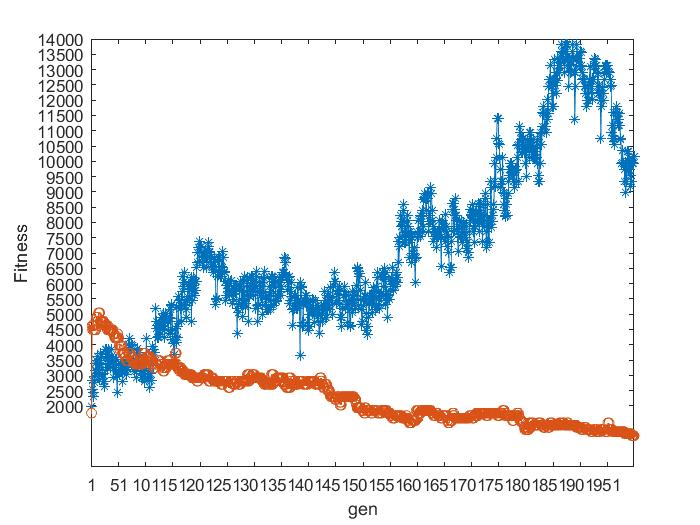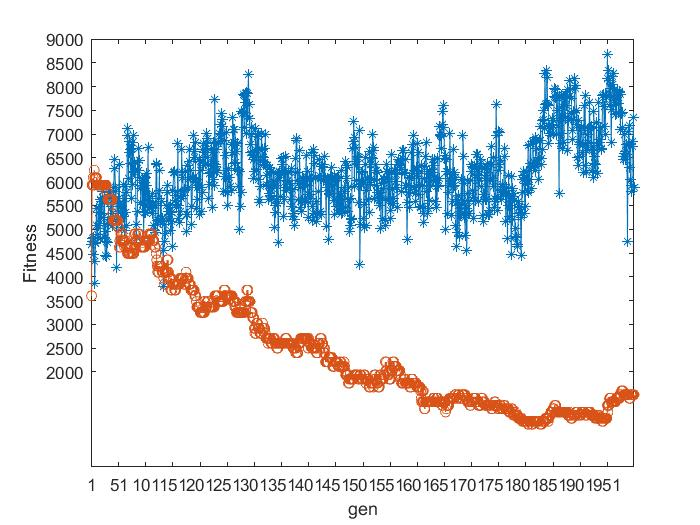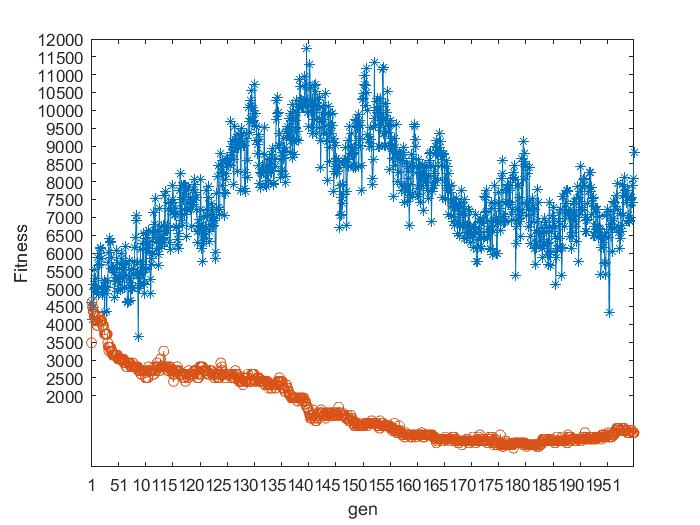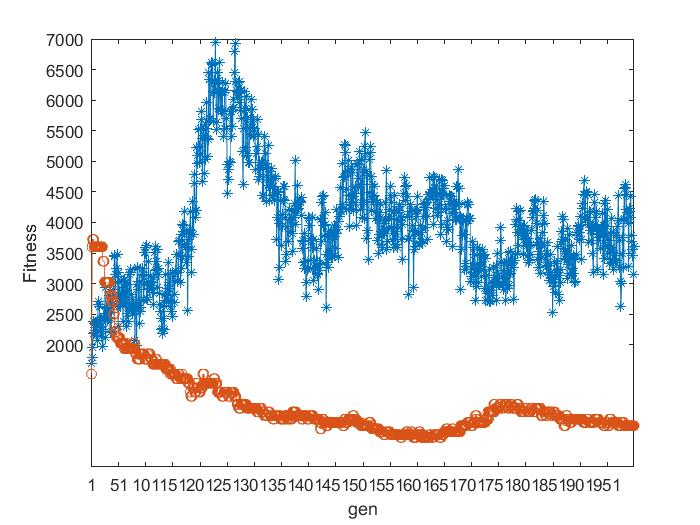可以看出二元锦标赛选择法是呈收敛趋势，轮盘赌选择算法效果较差，不呈现出收敛的趋势。其中该方法不能全局收敛的主要原因是由于存在统计误差，依据产生的随机数进行选择，有可能会出现不正确反应个体适应度的选择，可能导致适应度高的个体也被淘汰掉。    Rudolph已经采用有限马尔可夫链理论证明了仅采用交叉、变异和选择(轮盘赌选择法)三个遗传算子的标准遗传算法(Canonical Genetic Algorithm CGA)，不能收敛到全局最优值。由此需要算法需要引入一个重要的收敛策略-精英保留策略。 2. 个体精英保留策略与群体精英保留策略对比   个体精英保留策略是将父代中适应度最好的个体，不经过变异与交叉操作直接进入子代中，替换子代中适应度最差的个体。   群体精英保留策略是将父代种群和子代种群合并后，选择其中最优的N个（种群大小）个体构成下一代个体。下图为四个数据集基于轮盘赌选择方法采用不同的保留策略的测试结果。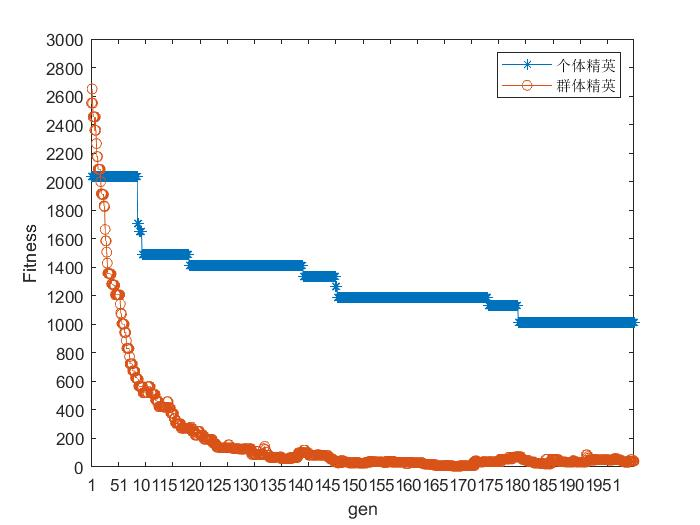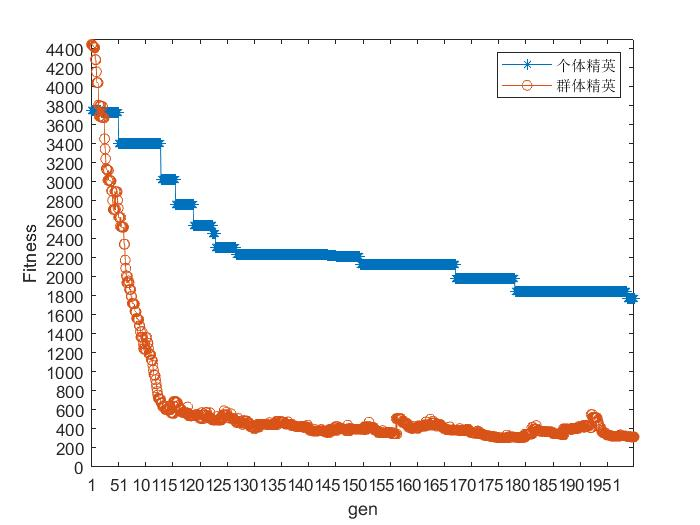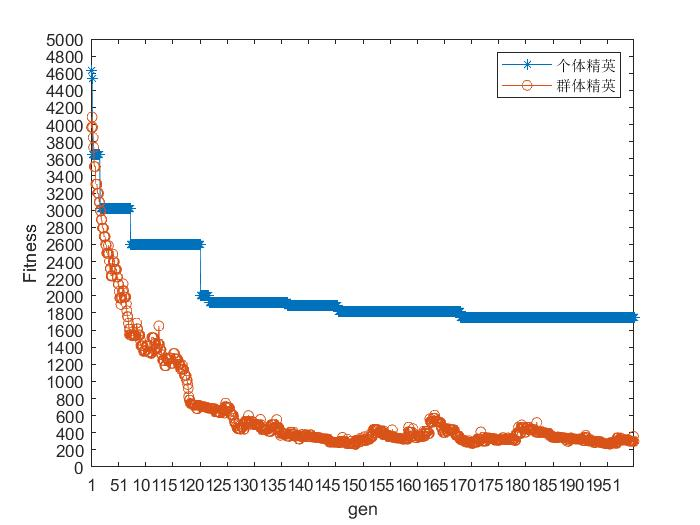在这里插入图片描述图中蓝色折线为个体精英保留策略，红色折线为种群精英保留策略，可以看出，
种群的精英保留策略收敛速度更快，相比个体精英策略更易于收敛到全局最优。
展开全文排课问题
• 　工程设计中最优化问题（optimalization problem）的一般提法是要选择一组参数（变量），在满足一系列有关的限制条件（约束）下，使设计指标（目标）达到最优值。因此，最优化问题通常可以表示为数学规划形式的问题...
• 目标优化实例和matlab程序 精品文档 精品文档 收集于网络如有侵权请联系管理员删除 收集于网络如有侵权请联系管理员删除 精品文档 收集于网络如有侵权请联系管理员删除 NSGA-II 算法实例 目前的多目标优化算法有很...
• 排课问题的本质是将课程、教师和学生在合适的时间段内分配到合适的教室中，涉及到的因素较，是一个多目标的调度问题，在运筹学中被称为时间表问题（Timetable Problem，TTP）。设一个星期有n个时段可排课，有m位...


C# 遗传算法 排课系统优化
一、要求二、内容三、分析流程图四、具体步骤五、理论基础1、遗传算法的科学定义2、遗传算法的执行过程
六、代码实现1、课程类2、算法实现3、界面设计
七、界面展示

本文将用C#语言来实现遗传算法对排课系统的优化，算法代码参考了洛荷大佬的Python实现基于遗传算法的排课优化，用C#实现后做了一个界面方便操作。

一、要求
导入学生（学号，姓名，性别），课程（课程号，课程时间，课容量），教师（教师工号，所教课程），教室（教室编号，教室容量）等基本信息；用数据初步处理，根据数据表建立老师与学生班的对应关系矩阵；用遗传算法对排课路径进行分析；对不同项目进行约束（教室容量满足性约束，同一教师同一时段上课不可冲突性约束，同一学生同一时段上课不可冲突性约束，任意课时使用教室数不超过学校教室的总量约束等约束）；求出群体中最大的适应值及其个体；设置罚值，淘汰不低质种群，求可行种群的目标函数值；计算目标函数（输出课程权值，教学资源的充分利用，可见学生流动量最小，相邻授课间隔尽量均匀，同一课程尽量只用一个教室等）。
二、内容
编写软件，实现界面友好的系统设计，完成整个排课系统优化的过程。 要求：每个步骤中，要把所有功能均编写成模块调用形式，如：导入数据，表间建立联 系、约束条件选择，排课课表导出与显示。课表按不同班级用户导出，用户可以根据提示进行选择，进入相应算法调用实现计算。
三、分析流程图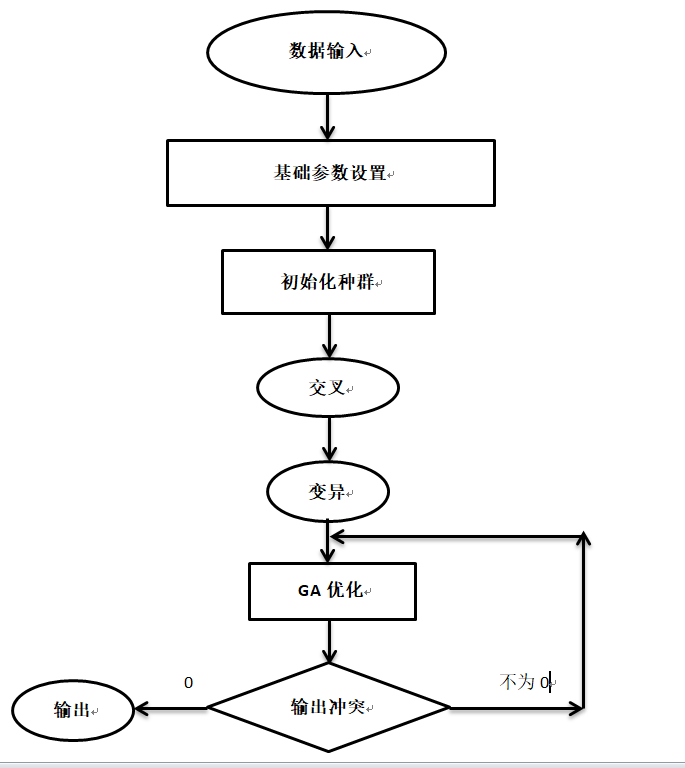四、具体步骤
1.导入数据并对数据进行初步处理； 2.设置基础参数：种群规模，突变的可能性，精英数目，执行次数。 3. 构造函数，初始化不同的种群（课程表的人数和教室数等）； 4.通过随机方式产生多个求解问题的二进制染色体编码，选择适应度高的染色体。 5. 交叉操作：随机对两个对象交换不同位置的属性，返回列表。交叉操作采用分块小基因交叉算法，即每个班的课程单元只能在相同的班级进行交叉操作，而不能跨班（行）进行交叉。例如两个个体进行交叉时，只能个体1的1班行基因与个体2的1班行基因进行交换。这样的操作不会破坏班级对课程和课时的要求。 6.变异操作：随机对Schedule象中对的某个可改变属性在允许范围内进行随机加减，返回列表，变异的过程是针对每个染色体个体内部的，为保证每个班级的既定的课程课时所以同交叉相同，变异操作限制在每个班级的基因段中。 7.GA优化：进化，启动GA算法进行优化，返回最佳结果的索引和最佳冲突的分数。计算课表种群的冲突，返回最佳结果的索引，最佳冲突的分数，当被测试课表冲突为0的时候，这个课表就是个符合规定的课表当冲突为零时，按班级输出当前排课表。
五、理论基础
1、遗传算法的科学定义
遗传算法（Genetic Algorithm, GA）是模拟达尔文生物进化论的自然选择和遗传学机理的生物进化过程的计算模型，是一种通过模拟自然进化过程搜索最优解的方法。
其主要特点是直接对结构对象进行操作，不存在求导和函数连续性的限定；具有内在的隐并行性和更好的全局寻优能力；采用概率化的寻优方法，不需要确定的规则就能自动获取和指导优化的搜索空间，自适应地调整搜索方向。
遗传算法以一种群体中的所有个体为对象，并利用随机化技术指导对一个被编码的参数空间进行高效搜索。其中，选择、交叉和变异构成了遗传算法的遗传操作；参数编码、初始群体的设定、适应度函数的设计、遗传操作设计、控制参数设定五个要素组成了遗传算法的核心内容。
2、遗传算法的执行过程
遗传算法是从代表问题可能潜在的解集的一个种群（population）开始的，而一个种群则由经过基因（gene）编码的一定数目的个体(individual)组成。每个个体实际上是染色体(chromosome)带有特征的实体。
染色体作为遗传物质的主要载体，即多个基因的集合，其内部表现（即基因型）是某种基因组合，它决定了个体的形状的外部表现，如黑头发的特征是由染色体中控制这一特征的某种基因组合决定的。因此，在一开始需要实现从表现型到基因型的映射即编码工作。由于仿照基因编码的工作很复杂，我们往往进行简化，如二进制编码。
初代种群产生之后，按照适者生存和优胜劣汰的原理，逐代（generation）演化产生出越来越好的近似解，在每一代，根据问题域中个体的适应度（fitness）大小选择（selection）个体，并借助于自然遗传学的遗传算子（genetic operators）进行组合交叉（crossover）和变异（mutation），产生出代表新的解集的种群。这个过程将导致种群像自然进化一样的后生代种群比前代更加适应于环境，末代种群中的最优个体经过解码（decoding），可以作为问题近似最优解。
六、代码实现
1、课程类
courseId代表课程号，classID代表班级号，teacherID代表教师号，这三个数据是从外部导入的，roomId代表教室号，weekDay代表星期，slot代表时间，这三个数据是随机生成的，通过遗传算法得到不冲突的结果。DeepClone()函数实现深度拷贝，RandomInit(int roomRange)生成随机的roomId,weekDay和slot。
[Serializable]
class Schedule : ICloneable
{
private int courseId;   //课程号
private int classId;    //班级号
private int teacherId;  //教师号
private int roomId = 0; //教室
private int weekDay = 0;    //星期
private int slot = 0;   //时间

public int CourseId { get => courseId; set => courseId = value; }
public int ClassId { get => classId; set => classId = value; }
public int TeacherId { get => teacherId; set => teacherId = value; }
public int RoomId { get => roomId; set => roomId = value; }
public int WeekDay { get => weekDay; set => weekDay = value; }
public int Slot { get => slot; set => slot = value; }

//构造函数
public Schedule(int courseId, int classId, int teacherId) //课程表类包含的内容，包括课程号，班级号，教师ID
{
this.courseId = courseId;
this.classId = classId;
this.teacherId = teacherId;
}

#region 拷贝主体
/// <summary>
/// 深度拷贝
/// </summary>
public Schedule DeepClone()
{
using (Stream objectStream = new MemoryStream())
{
IFormatter formatter = new BinaryFormatter();
formatter.Serialize(objectStream, this);
objectStream.Seek(0, SeekOrigin.Begin);
return formatter.Deserialize(objectStream) as Schedule;
}
}

public object Clone()
{
return this.MemberwiseClone();
}
#endregion

//随机匹配教室号和时间
public void RandomInit(int roomRange)
{
Random random = new Random();
this.RoomId = random.Next(1, roomRange + 1);
this.WeekDay = random.Next(1, 6);
this.Slot = random.Next(1, 6);  //随机生成时间
}

public override string ToString()
{
return String.Format("课程号：{0}\n班级号：{1}\n教师号：{2}\n房间号：{3}",CourseId,ClassId,TeacherId,RoomId);
}
}

2、算法实现
using System;
using System.Collections.Generic;
using System.Linq;
using System.Windows.Forms;

namespace Course_scheduling_optimization
{
class GeneticOptimize
{

private int popsize;   //种群规模
private double mutprob;   //突变的可能性
private int elite;  //精英数目
private int maxiter;  //执行次数
private Random random = new Random();   //随机数,方便调用函数

//封装字段
public int Popsize { get => popsize; set => popsize = value; }
public double Mutprob { get => mutprob; set => mutprob = value; }
public int Elite { get => elite; set => elite = value; }
public int Maxiter { get => maxiter; set => maxiter = value; }

//默认构造函数
public GeneticOptimize()
{
this.popsize = 30;
this.mutprob = 0.3;
this.elite = 5;
this.maxiter = 100;
}

//构造函数
public GeneticOptimize(int popsize, double mutprob, int elite, int maxiter)
{
this.popsize = popsize;
this.mutprob = mutprob;
this.elite = elite;
this.maxiter = maxiter;
}

//随机初始化不同的种群
//schedules：List,课程表的人数
//roomRange:int，教室数
private List<List<Schedule>> InitPopulation(List<Schedule> schedules,int roomRange)
{
List<List<Schedule>> population = new List<List<Schedule>>();
for (int i = 0; i < popsize; i++)
{
List<Schedule> entity = new List<Schedule>();
foreach (Schedule s in schedules)
{
s.RandomInit(roomRange);
}
}
return population;
}

//变异操作，随机对Schedule对象中的某个可改变属性在允许范围内进行随机加减，返回列表，变异后的种群
//eiltePopulation：List，精英时间表的种群
//roomRange: int,教室数
private List<Schedule> Mutate(List<List<Schedule>> eiltePopulation, int roomRange)
{
int e = random.Next(0, elite);  //elite-精英数目
int pos = random.Next(0, 2);
List<Schedule> ep = new List<Schedule>();    //再次生成Schedule对象
foreach (var epe in eiltePopulation[e])
{
}
foreach (var p in ep)
{
pos = random.Next(0, 3);
double operation = random.NextDouble();

if (pos == 0) p.RoomId = AddSub(p.RoomId, operation, roomRange);
if (pos == 1) p.WeekDay = AddSub(p.WeekDay, operation, 5);
if (pos == 2) p.Slot = AddSub(p.Slot, operation, 5);
}
return ep;
}

private int AddSub(int value,double op,int valueRange)
{
if(op > 0.5)
{
if (value < valueRange) value += 1;
else value -= 1;
}
else
{
if (value - 1 > 0) value -= 1;
else value += 1;
}
return value;
}

//交叉操作，随机对两个对象交换不同位置的属性，返回列表，交叉后的种群
//eiltePopulation:List,精英时间表的种群
private List<Schedule> Crossover(List<List<Schedule>> eiltePopulation)
{
int e1 = random.Next(0, elite);
int e2 = random.Next(0, elite);
int pos = random.Next(0, 2);

List<Schedule> ep1 = new List<Schedule>();
List<Schedule> ep2 = new List<Schedule>();

foreach (var epe1 in eiltePopulation[e1])
{
}

ep2 = eiltePopulation[e2];

for (int i = 0; i < ep1.Count; i++)
{
if(pos == 0)
{
ep1[i].WeekDay = ep2[i].WeekDay;
ep1[i].Slot = ep2[i].Slot;
}
if(pos == 1)
{
ep1[i].RoomId = ep2[i].RoomId;
}
}
return ep1;
}

//进化，启动GA算法进行优化，返回最佳结果的索引和最佳冲突的分数
//schedules:优化课程表
//elite：int，最佳结果的数目
public List<Schedule> evolution(List<Schedule> schedules, int roomRange, RichTextBox richTextBox)
{
//主循环
int bestScore = 0;
List<int> eliteIndex = new List<int>();
List<Schedule> newp = new List<Schedule>();
List<Schedule> bestSchedule = new List<Schedule>();
List<List<Schedule>> population = new List<List<Schedule>>();   //种群

population = InitPopulation(schedules, roomRange);   //初始化种群

for (int i = 0; i < maxiter; i++) //maxiter-执行次数
{
List<List<Schedule>> newPopulation = new List<List<Schedule>>();
Tuple<List<int>, int> scheduleCostRes = ScheduleCost(population, elite);//新的人口
eliteIndex = scheduleCostRes.Item1;  //精英指数
bestScore = scheduleCostRes.Item2;    //冲突最少的冲突数

richTextBox.Text += String.Format("Iter: {0} | conflict: {1}\n", i + 1, bestScore);
richTextBox.SelectionStart = richTextBox.TextLength;
richTextBox.ScrollToCaret();
//输出冲突

if (bestScore == 0)
{
richTextBox.Text += "排课完成";
bestSchedule = population[eliteIndex];
break;
}

//从精英开始
foreach (var ei in eliteIndex)
{
}

//添加精英的变异和繁殖形式
while(newPopulation.Count < popsize)
{

if (random.NextDouble() < mutprob)   //小于突变可能性
{
//突变
newp = Mutate(newPopulation, roomRange);   //变化
}
else
{
//交叉操作
newp = Crossover(newPopulation);
}
}
population = newPopulation;

if(i == maxiter - 1)
{
MessageBox.Show("未找到最佳排课结果！");
}
}
return bestSchedule;
}

// 计算课表种群的冲突，返回最佳结果的索引，最佳冲突的分数
// 当被测试课表冲突为0的时候，这个课表就是个符合规定的课表
// population:课程表的种群
// elite:最佳结果的数目
public Tuple<List<int>, int> ScheduleCost(List<List<Schedule>> population, int elite)
{
List<int> conflicts = new List<int>();
List<int> index = new List<int>();
List<int> bestResultIndex = new List<int>();
int n = population.Count;

foreach (List<Schedule> p in population)
{
int conflict = 0;
for (int i = 0; i < n - 1; i++)
{
for (int j = i + 1; j < n; j++)
{
//同一个教室在同一个时间只能有一门课
if (p[i].RoomId == p[j].RoomId & p[i].WeekDay == p[j].WeekDay & p[i].Slot == p[j].Slot)
conflict += 1;
//同一个班级在同一个时间只能有一门课
if (p[i].ClassId == p[j].ClassId & p[i].WeekDay == p[j].WeekDay & p[i].Slot == p[j].Slot)
conflict += 1;
//同一个教师在同一个时间只能有一门课
if (p[i].TeacherId == p[j].TeacherId & p[i].WeekDay == p[j].WeekDay & p[i].Slot == p[j].Slot)
conflict += 1;
//同一个班级在同一天不能有相同的课
if (p[i].ClassId == p[j].ClassId & p[i].CourseId == p[j].CourseId & p[i].WeekDay == p[j].WeekDay)
conflict += 1;
}
}
}
index = argsort(conflicts);   //返回列表值从小到大的索引值
for (int i = 0; i < elite; i++)
{
}
return Tuple.Create(bestResultIndex, conflicts[index]);
}

//argsort为手动实现Python中的numpy.argsort，返回列表值从小到大的索引值
public List<int> argsort(List<int> list)
{
Dictionary<int, int> indexDict = new Dictionary<int, int>();
List<int> indexList = new List<int>();
for (int i = 0; i < list.Count; i++)
{
}
var orderedDict = indexDict.OrderBy(x => x.Value).ToDictionary(x => x.Key, x => x.Value);
foreach (var key in orderedDict.Keys)
{
}
return indexList;
}
}
}


3、界面设计
using System;
using System.Windows.Forms;
using System.Collections.Generic;
using System.IO;
using System.Data;
using System.Linq;
using System.Drawing;

namespace Course_scheduling_optimization
{
public partial class Main : Form
{
private List<Schedule> res;  //全局变量

public Main()
{
InitializeComponent();
}

//限制textBox1只能输入正数
private void textBox1_KeyPress(object sender, KeyPressEventArgs e)
{
//如果输入的不是数字键，也不是回车键、Backspace键，则取消该输入
if (!(Char.IsNumber(e.KeyChar)) && (e.KeyChar != (char)13) && (e.KeyChar != (char)8))
{
e.Handled = true;
}
}

private void textBox3_KeyPress(object sender, KeyPressEventArgs e)
{
//如果输入的不是数字键，也不是回车键、Backspace键，则取消该输入
if (!(Char.IsNumber(e.KeyChar)) && (e.KeyChar != (char)13) && (e.KeyChar != (char)8))
{
e.Handled = true;
}
}

private void textBox5_KeyPress(object sender, KeyPressEventArgs e)
{
//如果输入的不是数字键，也不是回车键、Backspace键，则取消该输入
if (!(Char.IsNumber(e.KeyChar)) && (e.KeyChar != (char)13) && (e.KeyChar != (char)8))
{
e.Handled = true;
}
}

private void textBox6_KeyPress(object sender, KeyPressEventArgs e)
{
//如果输入的不是数字键，也不是回车键、Backspace键，则取消该输入
if (!(Char.IsNumber(e.KeyChar)) && (e.KeyChar != (char)13) && (e.KeyChar != (char)8))
{
e.Handled = true;
}
}

private void textBox4_KeyPress(object sender, KeyPressEventArgs e)
{
//如果输入的不是数字键，也不是回车键、Backspace键，也不是小数点，则取消该输入
if (((int)e.KeyChar < 48 || (int)e.KeyChar > 57) && (int)e.KeyChar != 8 && (int)e.KeyChar != 46)
e.Handled = true;

//小数点的处理。
if ((int)e.KeyChar == 46)                           //小数点
{
if (textBox4.Text.Length <= 0)
e.Handled = true;   //小数点不能在第一位
else
{
float f;
float oldf;
bool b1 = false, b2 = false;
b1 = float.TryParse(textBox4.Text, out oldf);
b2 = float.TryParse(textBox4.Text + e.KeyChar.ToString(), out f);
if (b2 == false)
{
if (b1 == true)
e.Handled = true;
else
e.Handled = false;
}
}
}
}

private void Main_Load(object sender, EventArgs e)
{
comboBox1.Items.Insert(0, "----请选择----");
comboBox1.SelectedIndex = 0;
//设置默认值
//textBox1.Text = "3";
textBox3.Text = "50";
textBox4.Text = "0.3";
textBox5.Text = "10";
textBox6.Text = "1000";

toolStripStatusLabel1.Text = "当前系统时间：" + DateTime.Now.ToString("yyyy-MM-dd hh:mm:ss");
this.timer1.Interval = 1000;
this.timer1.Start();

//IrisSkin4
skinEngine1.SkinFile = Application.StartupPath + @"/Skins/mp10.ssk";

}

//输出课程表
private void PrintSchedule(List<Schedule> res)
{
DataTable dt = new DataTable();
List<List<String>> Arr = new List<List<string>>();
for (int i = 1; i < 6; i++)
{
Arr.Add(new List<string>() { i.ToString(), "", "", "", "", "" });
}
//表头

foreach (var r in res)
{
int weekDay = r.WeekDay;
int slot = r.Slot;
Arr[slot-1][weekDay] = r.ToString();
}
foreach (var arr in Arr)
{
dt.Rows.Add(arr, arr, arr, arr, arr, arr);
}
dataGridView1.DataSource = dt;
//实现自动换行
dataGridView1.DefaultCellStyle.WrapMode = DataGridViewTriState.True;
dataGridView1.AutoSizeRowsMode = DataGridViewAutoSizeRowsMode.AllCells;
dataGridView1.AutoSizeColumnsMode = DataGridViewAutoSizeColumnsMode.Fill;
}

private void toolStripStatusLabel2_Click(object sender, EventArgs e)
{
toolStripStatusLabel2.Alignment = System.Windows.Forms.ToolStripItemAlignment.Right;
}

private void timer1_Tick(object sender, EventArgs e)
{
toolStripStatusLabel1.Text = "当前系统时间：" + DateTime.Now.ToString("yyyy-MM-dd hh:mm:ss");
}

private void button1_Click_1(object sender, EventArgs e)
{
//清空数据
richTextBox1.Text = "";
dataGridView1.DataSource = null;

if (textBox1.Text == "")
{
MessageBox.Show("请输入教室数目！");
}
else if (textBox2.Text == "")
{
MessageBox.Show("请选择文件路径！");
}
else
{
string filePath = textBox2.Text;
int roomRange = Convert.ToInt32(textBox1.Text);
int popsize = Convert.ToInt32(textBox3.Text);
double mutprob = Convert.ToDouble(textBox4.Text);
int elite = Convert.ToInt32(textBox5.Text);
int maxiter = Convert.ToInt32(textBox6.Text);

List<Schedule> schedules = new List<Schedule>();
List<int> classIds = new List<int>();

//清空数据
res = new List<Schedule>(); //全局变量初始化
comboBox1.Items.Clear();    //清空comboBox1中的数据
comboBox1.Items.Insert(0, "----请选择----");
comboBox1.SelectedIndex = 0;

//读入excel/csv文件
string line = "";
List<string[]> listStrArr = new List<string[]>();

while (line != null)
{

}
foreach (var s in listStrArr)
{
//放入schedule中
int courseId = Convert.ToInt32(s);
int classId = Convert.ToInt32(s);
int teacherId = Convert.ToInt32(s);
}

//优化
GeneticOptimize ga = new GeneticOptimize(popsize, mutprob, elite, maxiter);
res = ga.evolution(schedules, roomRange, richTextBox1);

//comboBox1绑定数据
foreach (var c in classIds.Distinct().ToList())
{
}
}
}

private void button2_Click(object sender, EventArgs e)
{
OpenFileDialog openFileDialog1 = new OpenFileDialog();  //显示选择文件对话框
openFileDialog1.InitialDirectory = "c:\\";
// openFileDialog1.Filter = "xlsx files (*.xlsx)|*.xlsx";
openFileDialog1.Filter = "csv files (*.csv)|*.csv";
openFileDialog1.FilterIndex = 2;
openFileDialog1.RestoreDirectory = true;

if (openFileDialog1.ShowDialog() == DialogResult.OK)
{
this.textBox2.Text = openFileDialog1.FileName;   //显示文件路径
//去textBox2.Text的路径下读取当前excel/csv文件
}
}

private void comboBox1_SelectedIndexChanged_2(object sender, EventArgs e)
{
if (comboBox1.SelectedIndex != 0)
{
int classId = Convert.ToInt32(comboBox1.Text);
List<Schedule> vis_res = new List<Schedule>();
foreach (var r in res)
{
if (r.ClassId == classId)
{
}
}
PrintSchedule(vis_res);
}
else
{
dataGridView1.DataSource = null;
}
}
}
}

七、界面展示
测试数据(保存为CSV文件）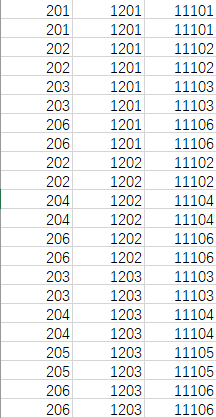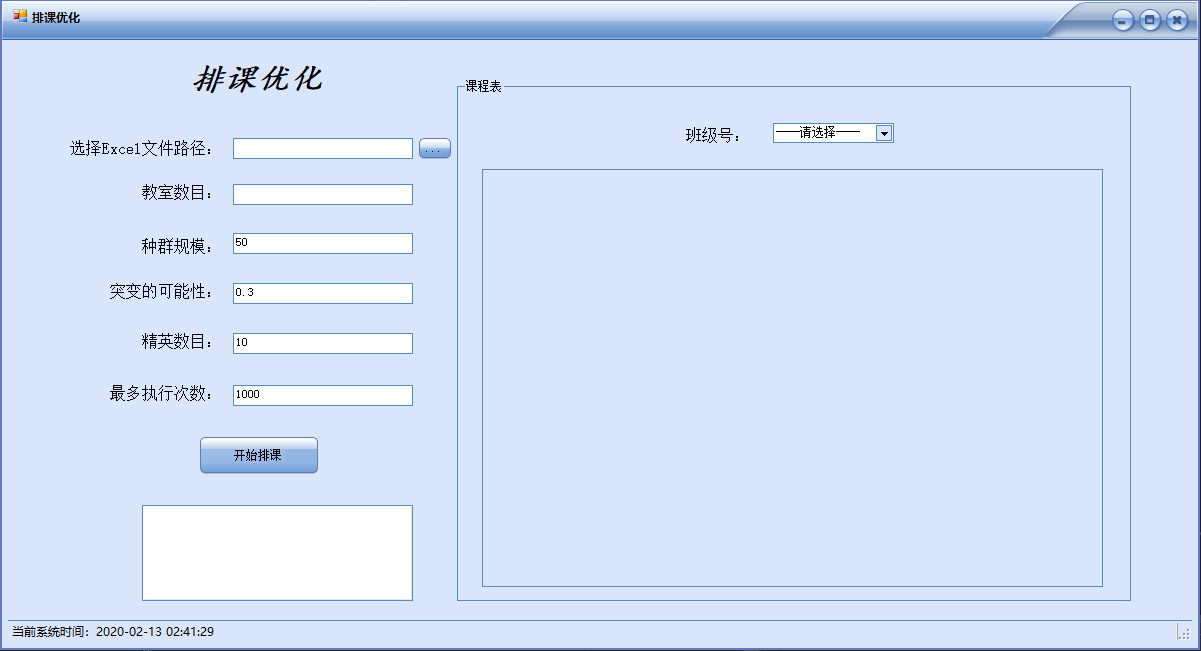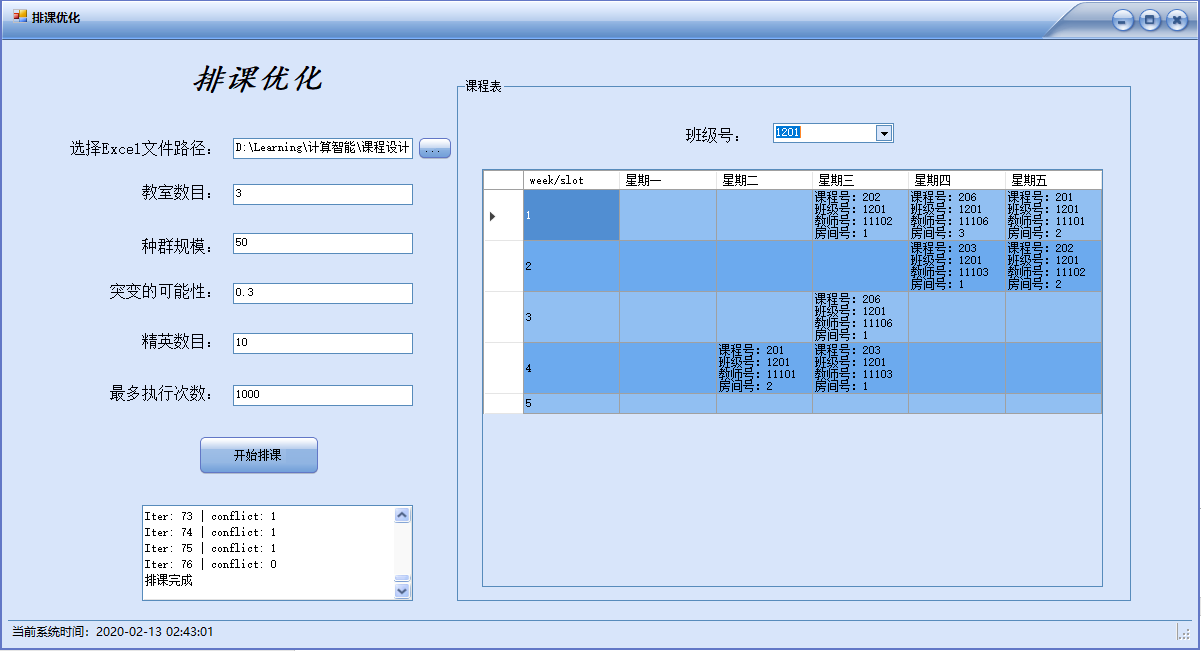展开全文c# 人工智能
• Pareto最优性，多目标优化算法 第十章 整数规划 . 混合整数规划算法概述，整数规划复杂性，搜索 第十一章 最优化方法在计算机算法中的应用 . 最优化方法在机器学习等领域的应用。 基本要求： 1、掌握最...
• ## 智能优化算法

万次阅读 多人点赞 2018-09-03 21:27:12
智能优化算法 目录 智能优化算法 目录 遗传算法（Genetic Algorithm） 理论 特点 领域 算法流程 差分进化算法（Differential Evolution Algorithm） 理论 特点 领域 算法流程 免疫算法（Immune Algorithm,...
• 原文引自Zhuoran Z , Changqiang H , Hanqiao H , et al. An optimization method:hummingbirds ...本文仅供自学和交流使用，未经允许请勿转载~谢谢Abstract: 本文介绍了一种优化算法 - 蜂鸟优化算法（HOA），...
• 智能优化算法——模拟退火算法 注：以下内容均由2017年黑龙江大学数学院暑期夏令营教学所用PPT总结 一．物理退火         为了更好地了解模拟退火算法，我们先从物理退火入手：     &...模拟退火算法 数学建模
• 智能优化算法：混合蛙跳算法 文章目录智能优化算法：混合蛙跳算法1.算法原理2.算法流程3.算法结果4.参考文献5.Matlab代码 摘要：混合蛙跳算法(SFLA)是Eusuff等人  于2003年提出的一种基于群体的亚启发式协同搜索...人工智能 机器学习
• 当前国内的运筹优化算法岗位行情是供不应求的。为什么会有这样的论断，难道博主没看到每年海内外各学校有相关专业大批硕博毕业生涌入市场么？有以下原因： 学术界留下了一部分优秀人才； 代码和工程能力较差； 国内...数据结构 深度学习
• J2ME优化压缩PNG文件 4个目标文件 内容索引:JAVA源码,综合应用,J2me游戏,PNG,图形处理 这是个J2ME控制台程序，它能剔除PNG文件中的非关键数据段，减少文件大小从而达到压缩图片的目的。而图片的质量并不会受到损失...java
• 基本程序协同进化遗传算法理论及应用》在详细阐述协同进化遗传算法原理与核心技术的同时，还给出其在多目标复杂数值函数优化机器人协调路径规划、神经网络结构与连接权值同时优化，以及群体决策中的具体应用...matlab
• 智能优化算法：萤火虫算法-附代码 文章目录智能优化算法：萤火虫算法-附代码1.算法原理2.算法结果3.参考文献4.Matlab代码 摘要：萤火虫算法（Fire-fly algorithm，FA）由剑桥大学 Yang 于 2009 年提出 , 作为最新的...python 人工智能 机器学习
• 个人总结迭代优化算法关键就两点： (1) 找到下降方向 (2) 确定下降步长 最速梯度下降算法 梯度下降算法是以最优化函数的梯度为下降方向，学习率ηη\eta乘以梯度的模即为下降步长。更新公式如下：xk+1...
• PO 是由巴基斯坦国立计算机和新兴科学大学的 Qamar Askari 等人于 2020 年提出的一种受社会启发的新型全局优化算法，灵感来源于政治的阶段过程。该算法通过将种群从逻辑上划分为政党和选区，赋予每个解双重角色，...
• PAGE 2 课程基本信息 课例编号 学科 信息技术 ...教学目标 教学目标 理解枚举算法的基本思想 认识问题解决过程中枚举算法的效率通过不同解题方法的比较体验算法优化合理选择算法 体验程序设计的基本过程通过对问题进
• 教学基于学习的优化是针对无约束问题的单目标优化技术。 在 TLBO 中，正如文献中所提出的，学生必须完成教师和学习者阶段。 接下来是经历教师和学生阶段的后续学生。 然而，在大多数实现中 [1,2]，所有成员都需要...matlab
• 文章目录概述前提条件设置学习率学习率的主流优化算作业 2-3 ...图1：“横纵式”教学法 — 优化算法 前提条件 在优化算法之前，需要进行数据处理、设计神经网络结构，代码与上一节保持一致，如下所示。...神经网络 python 深度学习
• 在Zitzler（一个巨佬）和K¨Unzli（2004，基于指标搜索的目标进化算法：IBEA）的带领下，人们对使用QI来指导多目标优化中的搜索越来越感兴趣。 Jiang等人（2015，一个简单而快速的基于HV超体积指标的目标进化...
• 本模型是基于极限学习机和遗传算法两种的优化和组合，并且得到了总合85%的预测成果。ELM和GA两模型的介绍如下： 极限学习机（Extreme LearningMachine,ELM）或“超限学习机”是一类基于前馈神经网络（Feedforward ...神经网络 机器学习 深度学习 python 数据挖掘
• morvan zhou教学视频https://morvanzhou.github.io/tutorials/machine-learning/reinforcement-learning/6-4-DPPO/ Hung-yi Lee课程http://speech.ee.ntu.edu.tw/~tlkagk/courses_MLDS18.html PPO论文...深度强化学习 人工智能 PPO...